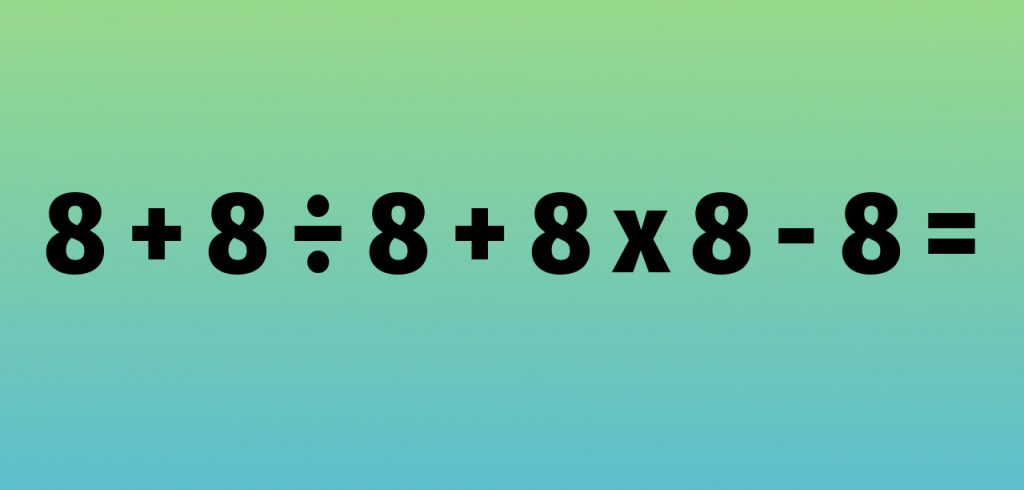Only 1 in 50 People Can Identify These 3 Math Mistakes. Can You?Only one out of fifty people can figure out these top three mistakes in mathematics. Can you figure them out?

Mistake #1: Order of Operations

What is the answer to 8 + 8 ÷ 8 + 8 x 8 – 8 = ?

Step 1. 8 + 8 ÷ 8 + 8 x 8 – 8 =
Step 2. 16 ÷ 8 + 8 x 8 – 8 =
Step 3. 2 + 8 x 8 – 8 =
Step 4. 10 x 8 – 8 =
Step 5. 80 – 8 = 72

If you said 72, you are wrong! There is a mistake in step 2 and in step 3.

The answer is actually 65 because the multiplication and division should be done before the addition and subtraction.

Step 1. 8 + 8 ÷ 8 + 8 x 8 – 8
Step 2. 8 + (8 ÷ 8) + (8 x 8) – 8
Step 3. 8 + 1 + 64 – 8 = 65 (Correct)

PEMDAS is Parenthesis, Exponents, Multiplication, Division, Addition, Subtraction. The order of the letters in PEMDAS tells you which part to calculate first. This is also known as the Order of Operations.

Mistake #2: Division by Zero

0 ÷ 2 = 0 so what is the answer to 2 ÷ 0 = ?

If you said 0, you are wrong!

The answer is actually undefined because you cannot divide by zero.

Step 2. Multiply both sides by a, so ab = a²
Step 3. Subtract b2 from both sides, so ab – b² = a² – b²
Step 4. Factor both sides, so b(a-b) = (a+b)(a-b)
Step 5. Divide both sides by a – b, so b = a + b
Step 6. Remember that a = b, so b = 2b
Step 7. Divide both sides by b, so 1 = 2

Obviously, one does not equal two! This shows the problems when you try to divide by zero. In step 5, a – b is zero because a = b. Therefore, there was a division by zero in this step.

Mistake #3: Forgotten Parentheses

What is the answer to (-4)² = ?

If you said negative 16 was the square of negative 4, you are wrong!

The answer is actually 16 not -16.

Here is an incorrect way to solve this problem: -4² = -(4)(4) = -16

And here is the correct way: (-4)² = (-4)(-4) = 16

The mistake is forgetting about the parentheses. Most people forget about the parentheses and just answer -16.